### Day 28 - Related Rates - 02.18.15

Update
• Unit 2b Test next Tuesday, 02.24

Bell Ringer

1.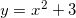Find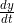when x = 1, given that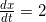when x = 1.

1. 0

2. 1

3. 2

4. 4

5. none of the above

2. A pebble is dropped into a calm pond, causing ripples in the form of concentric circles. The radius r of the outer ripple is increasing at a constant rate of 1 foot per second. When the radius is 4 feet, at what rate is the total area A of the disturbed water changing?

1. 8

2.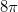3. 4

4.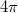5. none of the above

Review

Lesson
• Related Rates

Exit Ticket
• Posted on the board at the end of the block
Lesson Objectives
• How is implicit differentiation used to solve problems?

#### In-Class Help Requests

Standard(s)
• APC.5
• Investigate derivatives presented in graphic, numerical, and analytic contexts and the relationship between continuity and differentiability.
• The derivative will be defined as the limit of the difference quotient and interpreted as an instantaneous rate of change.
• APC.6
• ​The student will investigate the derivative at a point on a curve.
• Includes:
• finding the slope of a curve at a point, including points at which the tangent is vertical and points at which there are no tangents
• using local linear approximation to find the slope of a tangent line to a curve at the point
• ​defining instantaneous rate of change as the limit of average rate of change
• approximating rate of change from graphs and tables of values.
• APC.7
• Analyze the derivative of a function as a function in itself.
• Includes:
• comparing corresponding characteristics of the graphs of f, f', and f''
• ​defining the relationship between the increasing and decreasing behavior of f and the sign of f'
• ​translating verbal descriptions into equations involving derivatives and vice versa
• defining the relationship between the concavity of f and the sign of f "
• APC.9
• Apply formulas to find derivatives.
• Includes:
• derivatives of algebraic and trigonometric functions
• derivations of sums, products, quotients, inverses, and composites (chain rule) of elementary functions
• derivatives of implicitly defined functions
• higher order derivatives of algebraic and trigonometric functions

Past Checkpoints